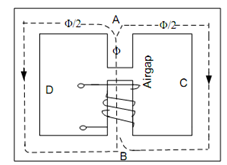## Series-Parallel Magnetic Circuit Assignment Help

Assignment Help: >> Parallel Magnetic Circuit - Series-Parallel Magnetic Circuit

Series-Parallel Magnetic Circuit:

After knowing series and parallel magnetic circuits, now we take example of a combination of the two. Such a circuit is illustrated in Figure. It contains two parallel magnetic circuits ACB and ADB connected across the common magnetic path AB which contains air gap of length lg. The flux φ in the common core is equally divided at point A among the two parallel paths which have equal reluctance. The reluctance of the path AB consists of (i) air gap reluctance, and (ii) the reluctance of the central core.Figure: Series-Parallel Magnetic Circuit